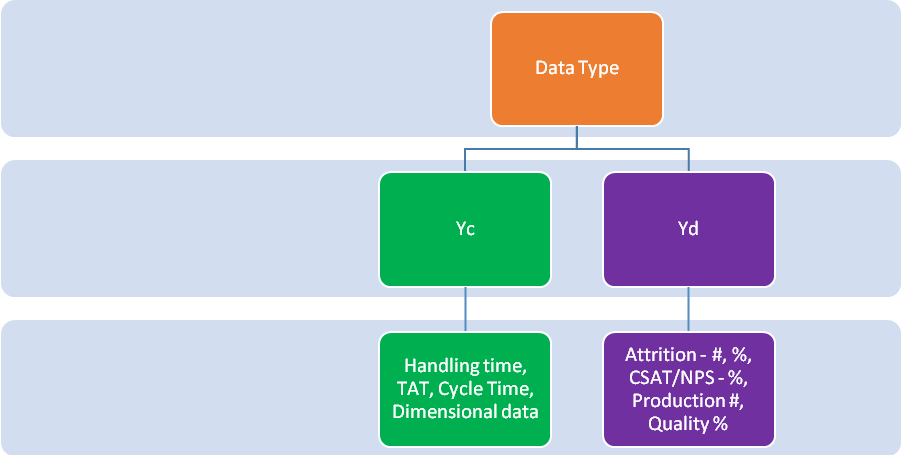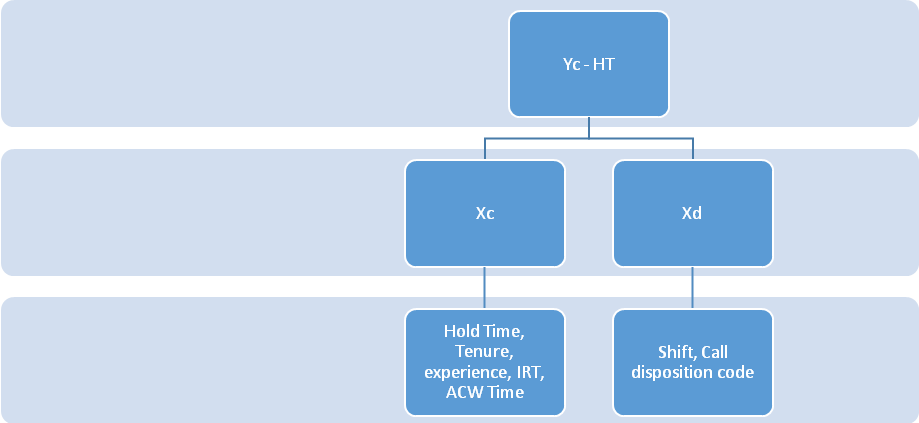## Lean Six Sigma | Analyze Phase

Problem Identification / IO Identification

Identified Stakeholders

Gather their Voice

Convert to CTQ

Create Project Charter

• Problem Statement / Opportunity Statement
• Goal Statement
• Scope Statement
• In Scope
• Out Scope
• Identify Team
• Define Roles and responsibilities
• RASIC/RACI/ ARMI
• Summary Milestone
• Detailed Flow Chart
• COPIS
• Benefits Documentation
• Risk Documentation

Measure

• Identify CTQ Metric
• Build DCP
• Collect Data
• Validated Data using MSA
• As-is Process Capability
• Numeric
• 1 Sided Spec
• Z (remember to transform data if data is non normal)
• 2 Sided Spec
• Z & Cp CPk (remember to transform data if data is non normal)
• Non-Numeric
• DPMO

Analyze

• Identify the potential factors (Xs)
• Brainstorming
• COPIS
• I stands for Input, Input shall be a key determinant of the outcome.
• Input & Resources must be evaluated as potential contributors
• Detailed Flowcharts
• Mathematical formulae and Derivatives
• Recruitment TAT = MPR + MPR Approval + Resume Shortlisting time + Interview TAT + Final Interview TAT + Offer Issuance TAT + Acceptance TAT….
• Study of Existing Data Strata
• Data wont exist in silo – it shall always have some other attributes that are captured with it.
• FMEA – Failure Mode and effect Analysis
• You try to find root case for the potential failure mode of the process

Analyze-1

1. Identify all Potential Xs

# Brainstorming – Reason of defects asks from all stakeholders

# Data Strata –

# Mathematical derivatives and formula

# I-Input of SIPOC/COPIS

#Process steps (detail flow chart)

# FMEA

1. Prepare DCP of Xs.
2. Collect Data of all Xs.
3. Understand behaviour of Y.
4. Check statistical relationship between Y&X.
5. Summary of analysis.

Next list down all the factors in excel and show all the factors in FISH bone. We will validate the factors whether one should be impacted or not through hypothesis testing/statistical relationship between Y & X.

• Build DCP for Factors
 Factor Name CTQ (Measurable Metric) Data Type Test to be used DVT Next Step Hold time # screens to toggle Process Knowledge

• Collect data for factors
• Perform hypothesis tests for statistical evidence of impact etc.

Hypothesis Testing (Assumption Testing): Method to check impact of X on Y is known as hypothesis testing.

Study the sample and pass the judgement

• Null Hypothesis
• Data is normal distributed
• Data is random( No significant evidence of the 4 patterns)
• Data is independent ( Y is not significantlyimpacted by X)
• Alternate Hypothesis
• Data is non-normal
• Data is non random
• Data is dependent ( Y is impacted by X)

Data has 3 basic characteristics

• Data is normal distributed
• If data were to adhere to the basic rules
• Mean +_1 Std Dev = 68%
• Mean +-2 Std Dev = 95%
• Mean +- 3 Std Dev = 99.74%
• If any of the three above rules are not met – we say that the data has evidence of special cause variation. (and process is referred to as out of control)
• Data is random (Run Chart)
• Path in Minitab – Stat > Quality tools > Run chart
• Absence of the 4 patterns in data is termed as randomness (Stability)
• Trend – Data is moving in one direction (either upwards or downwards).
• Cluster – Data which is close to each other.
• Some control is being exhibited to keep the data close to each other.
• Oscillation – To &Fro movement in data
• Ideally represents a upstream – downstream kind of process.
• Mixtures – Absence of data near the central line
• Represents 2 or more population in the same data.
• Data is independent
• All variables are independent of each other

P Value:

Decision criteria to choose between Ho & Ha is a value of probability referred to as P Value

Also referred to as value of significance – is a probability value (i.e. P value cannot be greater than 1 or 100% and cannot be smaller than 0 or 0%) and it represents contribution of chance in any relationship.

1 – Pvalue = Contribution of the factor

Cat causes accident.

Other factors (Chance) that could have caused accident – Rash driving / Drunk / Lubricant on the road / Someone else mistake

Salary causes attrition

Other factors that may have contributed is referred to as chance.

Cat experiment – P value - .12 (Chance)

Contribution of cat = 1-.12 = .88 or 88%

Salary impacting attrition

P Value = .01

Chance or other factors = 0.01

Salary = 1-.01 = .99

 Factor P Value Chance Factor Std = 95% Bad Boss .27 .27 1 - .27 = .73 or 73% Not impacting Salary .01 .01 .99 or 99% Impacting Growth Opp 0.03 .03 .97 or 97% Impacting Distance from office 0.0 0 100 Impacting

P Value < 0.05 = Ha will be accepted

P value = > 0.05 = Ho will be accepted.

LoHa

Confidence Level

The degree of surety about any inference.

Sample – Population

Population = 120 Cr

Sample =         12 Crore

1.2 Cr

12 Lakh

1.2 L

12000

Data Collection will have huge cost impact, may need too much time

Sample Size impact CL

Higher the sample size higher the Confidence Level

CL = 1- Alpha

95% CL – 5%

Minitab = 95% CL ( 5% Alpha error happening)

Alpha Error

Beta Error

 Pranay is innocent Free the guilty Pranay Beta Error Alpha Innocent Pranay is punished Pranay is guilty

When Ho is true but you accept Ha = Alpha error

When Ha is true and you accepted H0 – Beta error.

 Salary does not impact While salary impacts you have concluded it does not. Beta Error Alpha While salary does nt impact – you concluded that it did Salary impacts

Choice of Tests is dependent on data type of Y & XWhen Y is ContinuousWhen YC Xc

• Correlation
• Ho – Variables are independent
• Ha – Variables are related
• Step 1 : P Value received from the test
• Decide Ho or Ha
• St

DVT – Scatter Plot

YcXd – Regression Test

Step 1: P Value

• Ho : X does not significantly impact Y
• Ha : X significantly impacts Y

Step 2 : R Sq. – Coefficient of Determination – and it explains the % impact of X on Y

R Sq. = SSF/SST as %, industry standard for R Sq. = 62%, i.e. if R Sq. is greater than 62%, we consider the magnitude of impact as high.

Step 3 : If P is Ha, R Sqr is high – then check the regression equation ( also called transfer function)

Regression Analysis: Total Breakage versus Production(Kg)

The regression equation is

Total Breakage = 6.05 + 0.0198 Production(Kg)

Predictor           Coef   SE Coef     T      P

Constant           6.050     1.255  4.82  0.000

Production(Kg)  0.019802  0.004574  4.33  0.000

S = 2.47203   R-Sq = 8.3%   R-Sq(adj) = 7.9%

Analysis of Variance

Source           DF       SS      MS      F      P

Regression        1   114.53  114.53  18.74  0.000

Residual Error  206  1258.86    6.11

Total           207  1373.38

DVT – Scatter Plot